O 1 O O 1 O O 1 O 1 1 O 1 1 O 1 O 1 1 O O O O 1 O 1 1 O O 1 1 1 O 1 1 O 1 O O 1 O 1 1 O 1 1 1 O O 1 1 O O O O 1 O 1 1 1 O 1 O O O 1 1 O 1 O O 1 O 1 1 O 1 1 1 1 O 1 1 O 1 1 1 O O O 1 O O O O O O 1 1 O 1 O O 1 O 1 1 1 O O 1 1 O O 1 O O O O O O 1 1 O 1 1 O 1 O 1 1 O 1 1 1 1 O 1 1 1 O O 1 O O 1 1 O O 1 O 1 O O 1 O O O O O O 1 1 O 1 O O 1 O 1 1 O 1 1 O 1 O 1 1 1 O O O O O 1 1 O 1 1 1 1 O 1 1 1 O O 1 O O 1 1 1 O 1 O O O 1 1 O O O O 1 O 1 1 O 1 1 1 O O 1 1 1 O 1 O O O O 1 O O O O O O 1 1 1 O 1 O O O 1 1 O 1 O O O O 1 1 O O O O 1 O 1 1 O 1 1 1 O O O 1 O O O O O O 1 1 O 1 O 1 1 O 1 1 O 1 1 1 O O 1 1 O 1 1 1 1 O 1 1 1 O 1 1 1 O 1 1 O 1 1 O O O 1 1 O O 1 O 1 O 1 1 O O 1 O O O 1 1 O O 1 1 1 O 1 1 O O 1 O 1 O O 1 O 1 1 1 O O O 1 O O O O O O 1 O O O 1 1 O O 1 1 O 1 1 1 1 O 1 1 1 O O 1 O O O 1 O O O O O O 1 1 O 1 O 1 1 O 1 1 O 1 1 1 O O 1 1 O 1 1 1 1 O 1 1 1 O 1 1 1 O 1 1 O 1 1 O O O 1 1 O O 1 O 1 O 1 1 O O 1 O O O 1 1 O O 1 1 1 O 1 1 O O 1 O 1 O O 1 O O O O O O 1 1 O 1 O O 1 O 1 1 1 O O 1 1 O O 1 O O O O O O 1 1 O 1 1 O O O 1 1 O 1 O O 1 O 1 1 O 1 1 O 1 O 1 1 O 1 O O 1 O 1 1 1 O 1 O O O 1 1 O O 1 O 1 O 1 1 O O 1 O O O O 1 O O O O O O 1 1 1 O 1 O O O 1 1 O 1 1 1 1 O O 1 O O O O O O 1 1 O O O O 1 O 1 1 O 1 1 O O O 1 1 O 1 1 O O O O 1 O O O O O O 1 1 1 O 1 1 1 O 1 1 O O 1 O 1 O O 1 O O O O O O 1 1 O 1 1 1 O O 1 1 O 1 1 1 1 O 1 1 1 O 1 1 1 O O 1 O O O O O O 1 1 O 1 O 1 1 O 1 1 O 1 1 1 O O 1 1 O 1 1 1 1 O 1 1 1 O 1 1 1 O O 1 O O O O O O 1 1 O O O O 1 O 1 1 O 1 1 1 O O 1 1 O O 1 O O O O 1 O O O O O O 1 1 1 O 1 O 1 O 1 1 O 1 1 1 O O 1 1 O O 1 O O O 1 1 O O 1 O 1 O 1 1 1 O O 1 O O 1 1 1 O O 1 1 O 1 1 1 O 1 O O O 1 1 O O O O 1 O 1 1 O 1 1 1 O O 1 1 O O 1 O O O O 1 O 1 1 O O O O 1 O O O O O O 1 1 1 O 1 1 1 O 1 1 O 1 O O O O 1 1 O 1 O O 1 O 1 1 O 1 1 O O O 1 1 O O 1 O 1 O O 1 O O O O O O 1 1 O 1 O O 1 O 1 1 O 1 1 O 1 O 1 1 O O O O 1 O 1 1 O O 1 1 1 O 1 1 O 1 O O 1 O 1 1 O 1 1 1 O O 1 1 O O O O 1 O 1 1 1 O 1 O O O 1 1 O 1 O O 1 O 1 1 O 1 1 1 1 O 1 1 O 1 1 1 O O O 1 O O O O O O 1 1 O O 1 O 1 O 1 1 O 1 1 O 1 O 1 1 O O O 1 O O 1 1 1 O O 1 O O 1 1 O O O O 1 O 1 1 O O O 1 1 O 1 1 O O 1 O 1 O 1 1 1 O O 1 1 O O 1 O O O O O O 1 1 1 O 1 O O O 1 1 O 1 O O O O 1 1 O O 1 O 1 O O 1 O O O O O O 1 1 O O 1 O 1 O 1 1 O 1 1 1 O O 1 1 1 O 1 O O O 1 1 O 1 O O 1 O 1 1 1 O O 1 O O 1 1 O O 1 O 1 O O 1 O O O O O O 1 1 1 O 1 1 1 O 1 1 O 1 1 1 1 O 1 1 1 O O 1 O O 1 1 O 1 1 O O O 1 1 O O 1 O O O O 1 O 1 1 O O O O 1 O O O O O O 1 1 O O O O 1 O 1 1 O 1 1 1 O O 1 1 O O 1 O O O O 1 O O O O O O 1 1 O O O O 1 O 1 1 O 1 1 O O O 1 1 O 1 1 O O O O 1 O O O O O O 1 1 1 O 1 O O O 1 1 O 1 O O O O 1 1 O O 1 O 1 O 1 1 1 O O 1 O O 1 1 O O 1 O 1 O O 1 O O O O O O 1 1 O O 1 O 1 O 1 1 1 O 1 1 O O 1 1 O O 1 O 1 O 1 1 1 O O 1 O O O 1 O O O O O O 1 1 1 O 1 1 1 O 1 1 O 1 O O 1 O 1 1 O 1 1 O O O 1 1 O 1 1 O O O O 1 O O O O O O 1 1 O O O 1 O O 1 1 O O 1 O 1 O O 1 O O O O O O 1 1 1 O 1 O O O 1 1 O 1 1 1 1 O O 1 O O O O O O 1 1 O 1 O 1 1 O 1 1 O 1 1 1 O O 1 1 O 1 1 1 1 O 1 1 1 O 1 1 1 O O 1 O O O O O O 1 1 O O O O 1 O 1 1 O 1 1 1 O O 1 1 O O 1 O O O O 1 O O O O O O 1 1 1 O 1 O 1 O 1 1 O 1 1 1 O O 1 1 O O 1 O O O 1 1 O O 1 O 1 O 1 1 1 O O 1 O O 1 1 1 O O 1 1 O 1 1 1 O 1 O O O 1 1 O O O O 1 O 1 1 O 1 1 1 O O 1 1 O O 1 O O
My Widgets# 链接

Reviews : appgefahren.de, iphon.fr, mobile.softpedia.com (4.5/5), antyapps.pl (4+), ioshacker.com, gizmodo.co.uk, iapp-mac.com, idevice.ro, iphoneitalia.com

# 视频

 (v2.0 on iPhone 6) (v2.0 on iPad)

# iPhone截图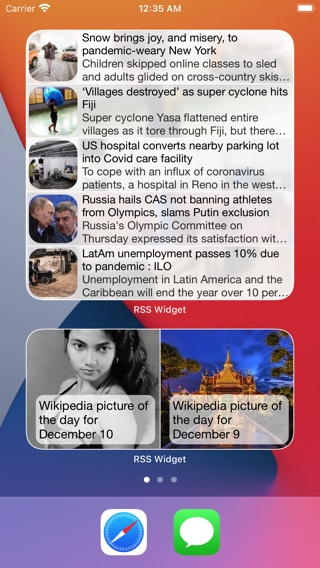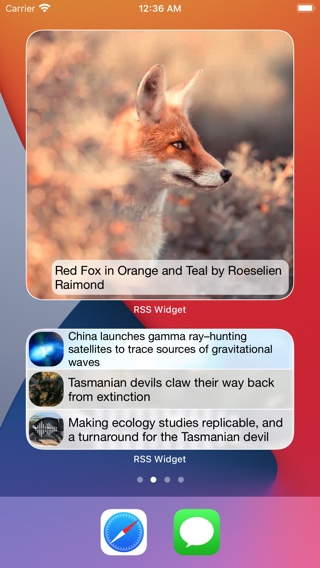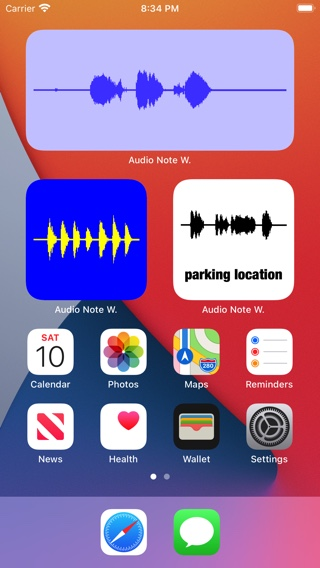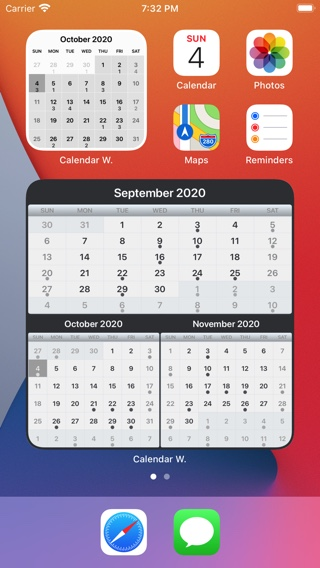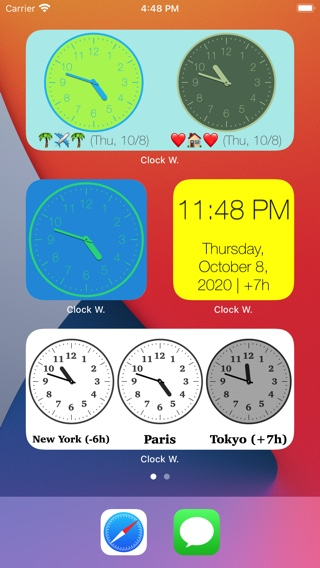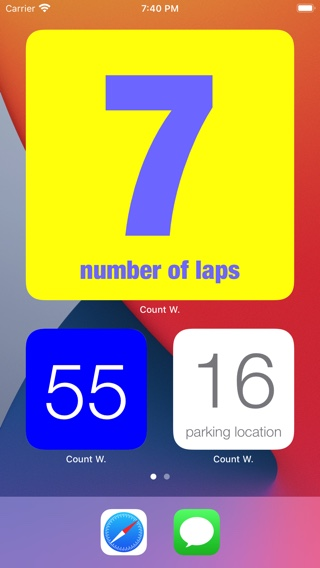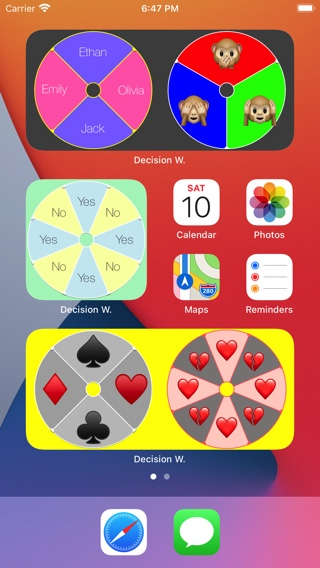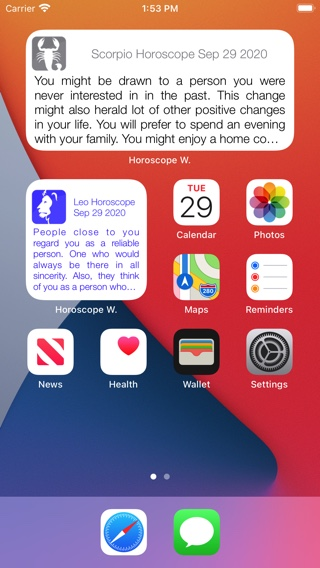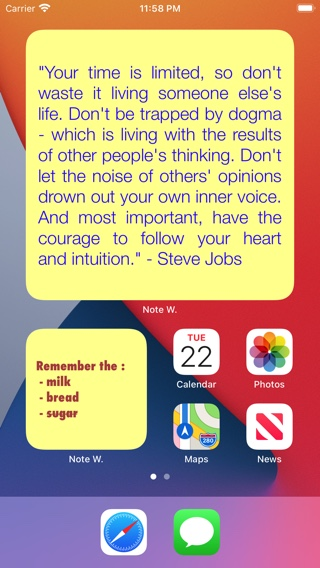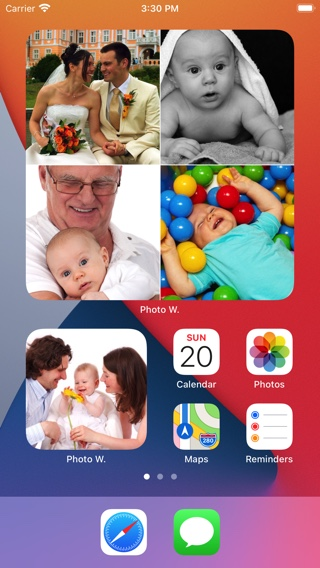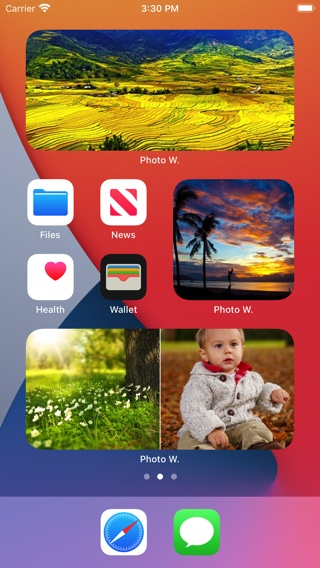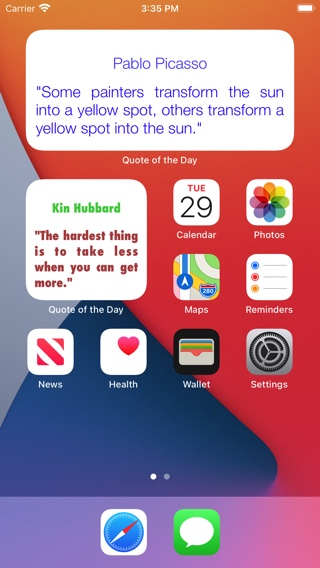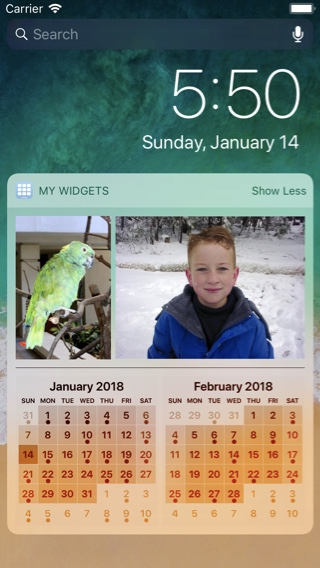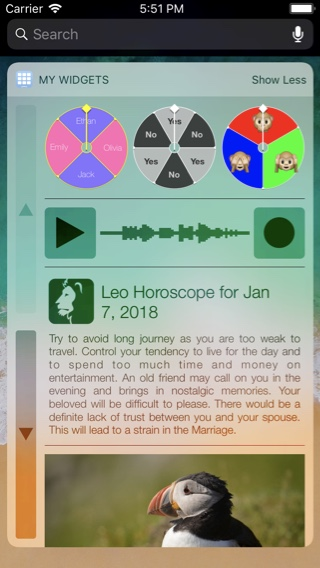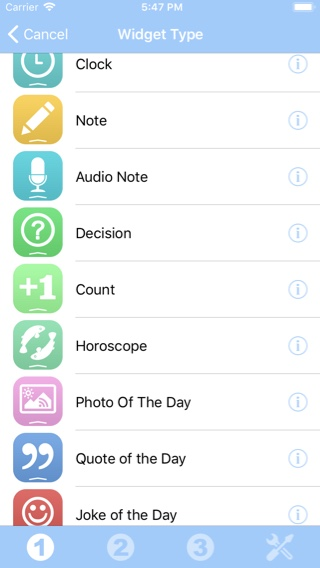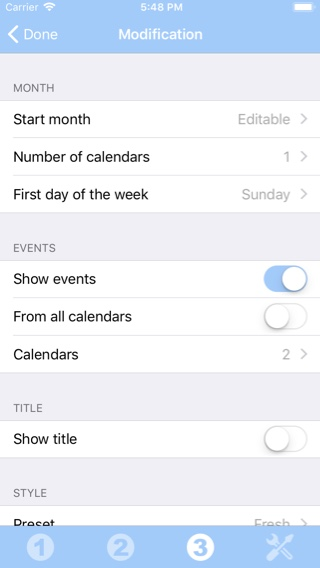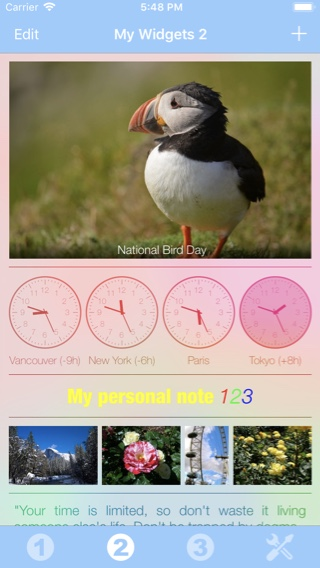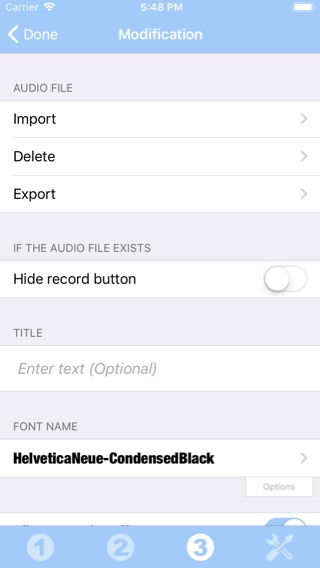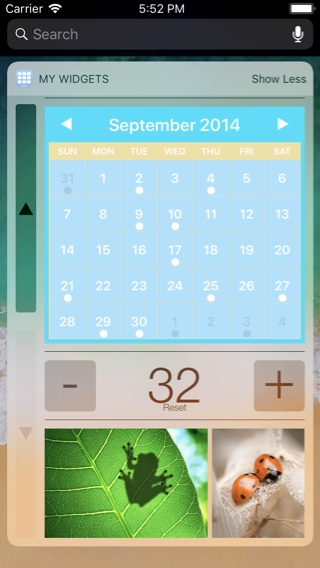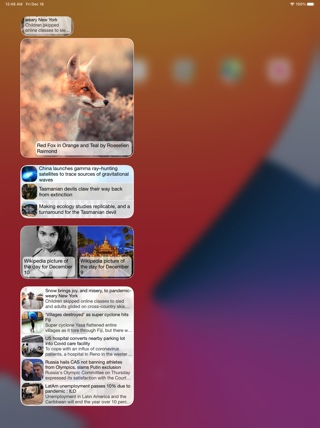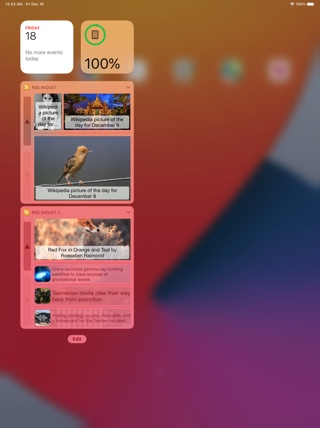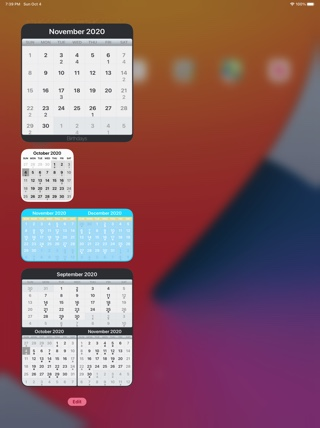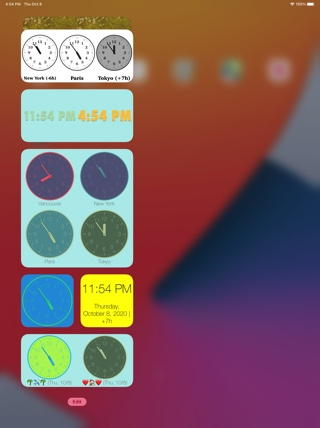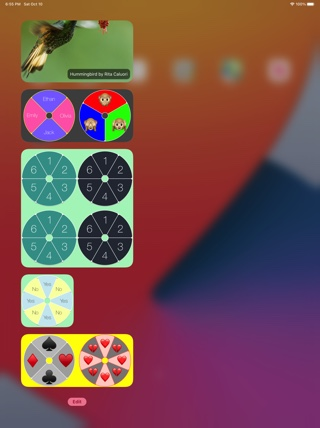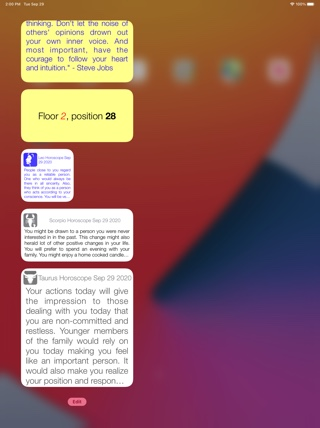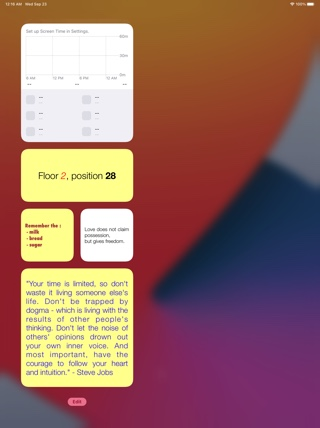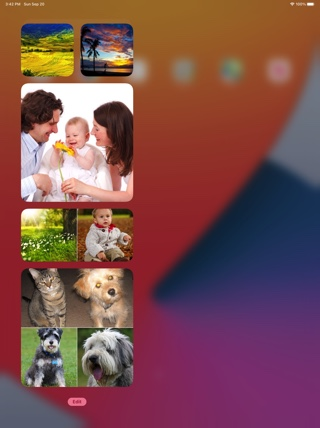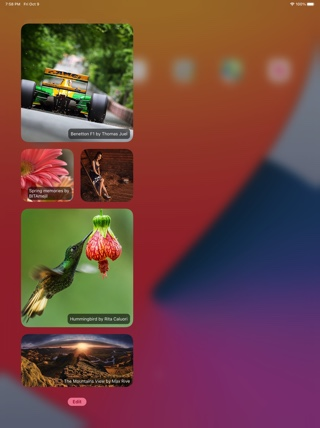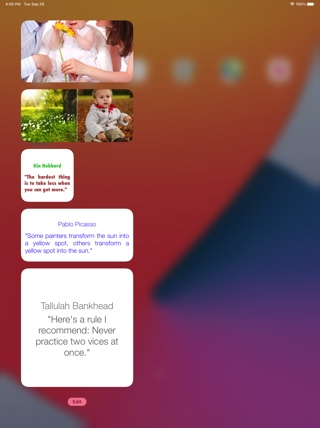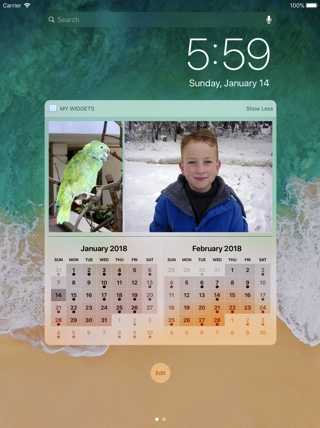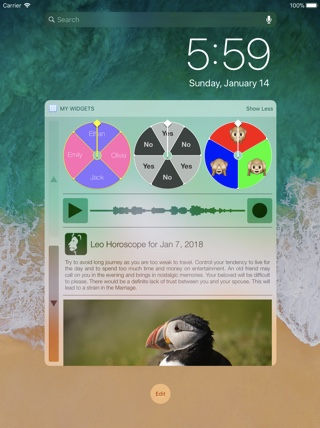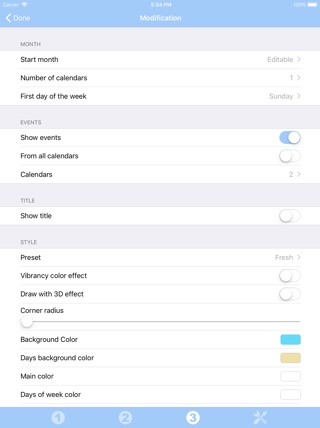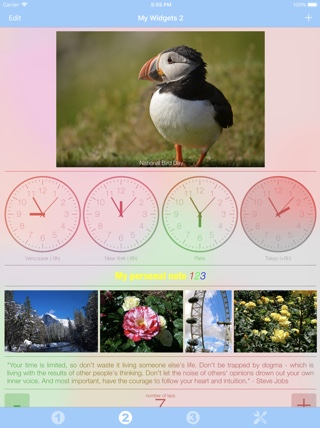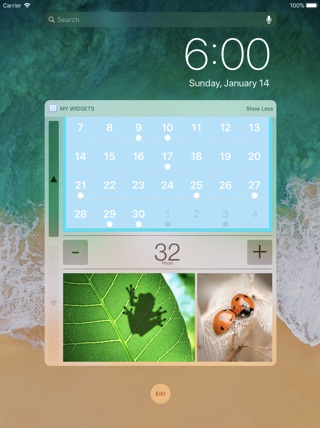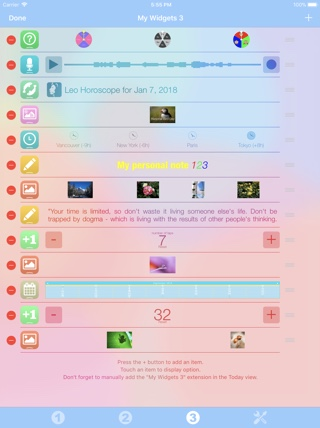# 历史

## v5.3

• Internet content: Possibility to open the URL of a widget in the internal web browser of the application (without distractions) instead of opening it in Safari.
• Internet content: Possibility to display the image in full screen, instead of opening the URL of the page.
• News: new sources of news.
• Photo: possibility to crop the photo during import.

# 可用性